# Introduction to BDgraph

#### 2022-12-24

This introduction to the R package BDgraph is a modified version of Mohammadi and Wit (2019), published in the Journal of Statistical Software.

The R package BDgraph provides statistical tools for Bayesian structure learning for undirected graphical models with continuous, count, binary, and mixed data. The package is implemented the recent improvements in the Bayesian graphical models’ literature, including Mohammadi and Wit (2015), Mohammadi et al. (2021), Mohammadi et al. (2017), Dobra and Mohammadi (2018), and Vinciotti et al. (2022). Besides, the package contains several functions for simulation and visualization, as well as several multivariate datasets taken from the literature.

# 1 User interface

In the R environment, one can access and load the BDgraph package by using the following commands:

Install BDgraph using

install.packages( "BDgraph" )

library( BDgraph )

To speed up computations, we efficiently implement the BDgraph package by linking the C++ code to R. The computationally extensive tasks of the package are implemented in parallel in C++ using OpenMP. For the C++ code, we use the highly optimized LAPACK and BLAS, as linear algebra libraries on systems that provide them. The use of these libraries significantly improves program speed.

We design the BDgraph package to provide a Bayesian framework for undirected graph estimation of different types of datasets such as continuous, discrete or mixed data. The package facilitates a pipeline for analysis by four functional modules as follows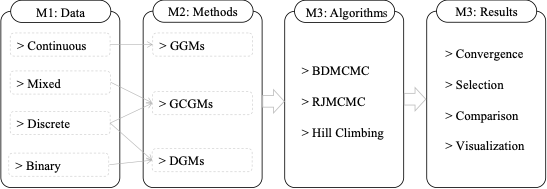Module 1. Data simulation: Function bdgraph.sim() simulates multivariate Gaussian, discrete, binary, and mixed data with different undirected graph structures, including “random”, “cluster”, “scale-free”, “lattice”, “hub”, “star”, “circle”, “AR(1)”, “AR(2)”, and “fixed” graphs. Users can determine the sparsity of the graph structure and can generate mixed data, including “count”, “ordinal”, “binary”, “Gaussian”, and “non-Gaussian” variables.

Module 2. Methods: The function bdgraph(), bdgraph.mpl(), and bdgraph.dw() provide several estimation methods regarding to the type of data:

• Bayesian graph estimation for the multivariate data that follow the Gaussianity assumption, based on the Gaussian graphical models (GGMs); see Mohammadi and Wit (2015).
• Bayesian graph estimation for multivariate non-Gaussian, discrete, and mixed data, based on Gaussian copula graphical models (GCGMs); see Mohammadi et al. (2017) and Vinciotti et al. (2022).
• Bayesian graph estimation for multivariate discrete and binary data, based on discrete graphical models (DGMs); see Dobra and Mohammadi (2018).

Module 3. Algorithms: The function bdgraph() and bdgraph.mpl() provide several sampling algorithms:

Module 4. Results: Includes four types of functions:

• Graph selection: The functions select(), plinks(), and pgraph() provide the selected graph, the posterior link inclusion probabilities and the posterior probability of each graph, respectively.
• Convergence check: The functions plotcoda() and traceplot() provide several visualization plots to monitor the convergence of the sampling algorithms.
• Comparison and goodness-of-fit: The functions compare() and plotroc() provide several comparison measures and an ROC plot for model comparison.
• Visualization: The plotting functions plot.bdgraph() and plot.sim() provide visualizations of the simulated data and estimated graphs.

# 2 The BDgraph environment

The BDgraph package provides a set of comprehensive tools related to Bayesian graphical models; we describe below the essential functions available in the package.

## 2.1 Posterior sampling

We design the function bdgraph(), as the main function of the package, to take samples from the posterior distributions based on both of our Bayesian frameworks (GGMs and GCGMs). By default, the bdgraph() function is based on underlying sampling algorithm defined in Mohammadi et al. (2021). Moreover, as an alternative to those BDMCMC sampling algorithms, we implement RJMCMC sampling algorithms for both the Gaussian and non-Gaussian frameworks. By using the following function

bdgraph( data, n = NULL, method = "ggm", algorithm = "bdmcmc", iter = 5000,
burnin = iter / 2, not.cont = NULL, g.prior = 0.5, df.prior = 3,
g.start = "empty", jump = NULL, save = FALSE,
cores = NULL, threshold = 1e-8, verbose = TRUE )

we obtain a sample from our target joint posterior distribution. bdgraph() returns an object of S3 class type bdgraph. The functions plot(), print(), and summary() are working with the object bdgraph. The input data can be an ($$n \times p$$) matrix or a data.frame or a covariance ($$p \times p$$) matrix ($$n$$ is the sample size and $$p$$ is the dimension); it can also be an object of class sim, which is the output of function bdgraph.sim().

The argument method determines the type of methods, GGMs, GCGMs. Option “ggm” is based on Gaussian graphical models that is designed for multivariate Gaussian data. Option “gcgm” is based on the GCGMs that is designed for non-Gaussian data such as, non-Gaussian continuous, discrete or mixed data.

The argument algorithm refers the type of sampling algorithms which could be based on BDMCMC or RJMCMC. Option “bdmcmc” (as default) is for the BDMCMC sampling algorithms. Option “rjmcmc” is for the RJMCMC sampling algorithms, which are alternative algorithms. See Mohammadi and Wit (2015).

The argument g.start specifies the initial graph for our sampling algorithm. It could be “empty” (default) or “full”. Option “empty” means the initial graph is an empty graph and “full” means a full graph. It also could be an object with S3 class , which allows users to run the sampling algorithm from the last objects of the previous run.

The argument jump determines the number of links that are simultaneously updated in the BDMCMC algorithm.

For parallel computation in C++ which is based on OpenMP, user can use argument cores which specifies the number of cores to use for parallel execution.

Note, the package BDgraph has two other sampling functions, bdgraph.mpl() and bdgraph.dwl() which are designed in the similar framework as the function bdgraph(). The function bdgraph.mpl() is for Bayesian model determination in undirected graphical models based on marginal pseudo-likelihood, for both continuous and discrete variables; For more details see . The function bdgraph.dwl() is for Bayesian model determination for count data; See Vinciotti et al. (2022).

## 2.2 Posterior graph selection

We design the BDgraph package in such a way that posterior graph selection can be done based on both Bayesian model averaging (BMA), as default, and maximum a posterior probability (MAP). The functions select() and plinks() are designed for the objects of class bdgraph to provide BMA and MAP estimations for posterior graph selection.

The function

plinks( bdgraph.obj, round = 2, burnin = NULL )

provides estimated posterior link inclusion probabilities for all possible links, which is based on BMA estimation. In cases where the sampling algorithm is based on BDMCMC, these probabilities for all possible links $$e=(i,j)$$ in the graph can be estimated using a Rao-Blackwellized estimate based on $\begin{eqnarray} \label{posterior-link} Pr( e \in E | data )= \frac{\sum_{t=1}^{N}{1(e \in E^{(t)}) W(K^{(t)}) }}{\sum_{t=1}^{N}{W(K^{(t)})}}, \end{eqnarray}$ where $$N$$ is the number of iteration and $$W(K^{(t)})$$ are the weights of the graph $$G^{(t)}$$ with the precision matrix $$K^{(t)}$$.

The function

select( bdgraph.obj, cut = NULL, vis = FALSE )

provides the inferred graph based on both BMA (as default) and MAP estimators. The inferred graph based on BMA estimation is a graph with links for which the estimated posterior probabilities are greater than a certain cut-point (as default cut=0.5). The inferred graph based on MAP estimation is a graph with the highest posterior probability.

Note, for posterior graph selection based on MAP estimation we should save all adjacency matrices by using the option save = TRUE in the function bdgraph(). Saving all the adjacency matrices could, however, cause memory problems.

## 2.3 Convergence check

In general, convergence in MCMC approaches can be difficult to evaluate. From a theoretical point of view, the sampling distribution will converge to the target joint posterior distribution as the number of iteration increases to infinity. Because we normally have little theoretical insight about how quickly MCMC algorithms converge to the target stationary distribution we therefore rely on post hoc testing of the sampled output. In general, the sample is divided into two parts: a burn-in’’ part of the sample and the remainder, in which the chain is considered to have converged sufficiently close to the target posterior distribution. Two questions then arise: How many samples are sufficient? How long should the burn-in period be?

The plotcoda() and traceplot() are two visualization functions for the objects of class bdgraph that make it possible to check the convergence of the search algorithms in BDgraph. The function

plotcoda( bdgraph.obj, thin = NULL, control = TRUE, main = NULL,
verbose = TRUE, ... )

provides the trace of estimated posterior probability of all possible links to check convergence of the search algorithms. Option is designed for the case where if control=TRUE (as default) and the dimension ($$p$$) is greater than $$15$$, then $$100$$ links are randomly selected for visualization.

The function

traceplot( bdgraph.obj, acf = FALSE, pacf = FALSE, main = NULL, ... )

provides the trace of graph size to check convergence of the search algorithms. Option acf is for visualization of the autocorrelation functions for graph size; option pacf visualizes the partial autocorrelations.

## 2.4 Comparison and goodness-of-fit

The functions compare() and plotroc() are designed to evaluate and compare the performance of the selected graph. These functions are particularly useful for simulation studies. With the function

compare( pred, actual, main = NULL, vis = FALSE ) 

we can evaluate the performance of the Bayesian methods available in our BDgraph package and compare them with alternative approaches. This function provides several measures such as the balanced $$F$$-score measure, which is defined as follows: $\begin{eqnarray} \label{f1} F_1\mbox{-score} = \frac{2 \mbox{TP}}{2 \mbox{TP + FP + FN}}, \end{eqnarray}$ where TP, FP and FN are the number of true positives, false positives and false negatives, respectively. The $$F_1$$-score lies between $$0$$ and $$1$$, where $$1$$ stands for perfect identification and $$0$$ for no true positives.

The function

plotroc = function( pred, actual, cut = 20, smooth = FALSE, ... )

provides a ROC plot for visualization comparison based on the estimated posterior link inclusion probabilities. See also function roc() for a ROC curve specifically for graph structure learning.

## 2.5 Data simulation

The function bdgraph.sim() is designed to simulate different types of datasets with various graph structures. The function

bdgraph.sim( p = 10, graph = "random", n = 0, type = "Gaussian", prob = 0.2,
size = NULL, mean = 0, class = NULL, cut = 4, b = 3,
D = diag( p ), K = NULL, sigma = NULL,
q = exp(-1), beta = 1, vis = FALSE, rewire = 0.05,
range.mu = c( 3, 5 ), range.dispersion = c( 0.01, 0.1 ) )

can simulate multivariate Gaussian, non-Gaussian, discrete, binary and mixed data with different undirected graph structures, including “random”, “cluster”, “scale-free”, “lattice”, “hub”, “star”, “circle”, “AR(1)”, “AR(2)”, and “fixed” graphs. Users can specify the type of multivariate data by option type and the graph structure by option graph. They can determine the sparsity level of the obtained graph by using option prob. With this function users can generate mixed data from “count”, “ordinal”, “binary”, “Gaussian” and “non-Gaussian” distributions. bdgraph.sim() returns an object of the S3 class type “sim”. Functions plot() and print() work with this object type.

There is another function in the BDgraph package with the name graph.sim() which is designed to simulate different types of graph structures. The function

graph.sim( p = 10, graph = "random", prob = 0.2, size = NULL, class = NULL,
vis = FALSE, rewire = 0.05 )

can simulate different undirected graph structures, including “random”, “cluster”, “scale-free”, “lattice”, “hub”, “star”, and “circle” graphs. Users can specify the type of graph structure by option graph. They can determine the sparsity level of the obtained graph by using option prob. bdgraph.sim() returns an object of the S3 class type “graph”. Functions plot() and print() work with this object type.

# 3 An example on simulated data

We illustrate the user interface of the BDgraph package by use of a simple simulation. By using the function bdgraph.sim() we simulate $$60$$ observations ($$n=60$$) from a multivariate Gaussian distribution with $$8$$ variables ($$p=8$$) and scale-free’’ graph structure, as below.

library( BDgraph )

set.seed( 5 )

data.sim <- bdgraph.sim( n = 60, p = 8, graph = "scale-free", type = "Gaussian" )
round( head( data.sim $data, 4 ), 2 ) [,1] [,2] [,3] [,4] [,5] [,6] [,7] [,8] [1,] 0.93 -1.50 -1.77 -0.31 0.17 -0.70 0.17 0.90 [2,] 0.32 -0.18 0.13 1.00 0.08 -0.06 -0.29 -0.56 [3,] -0.54 -0.15 -0.40 -0.80 1.72 1.24 -1.79 1.05 [4,] -0.43 -0.81 0.98 -2.50 -0.97 0.37 -0.80 2.52 Since the generated data are Gaussian, we run the BDMCMC algorithm which is based on Gaussian graphical models. For this we choose method = "ggm", as follows: sample.bdmcmc <- bdgraph( data = data.sim, method = "ggm", algorithm = "bdmcmc", iter = 5000, save = TRUE, verbose = FALSE ) We choose option save = TRUE to save the samples in order to check convergence of the algorithm. Running this function takes less than one second, as the computational intensive tasks are performed in C++ and interfaced with R. Since the function bdgraph() returns an object of class S3, users can see the summary result as follows summary( sample.bdmcmc )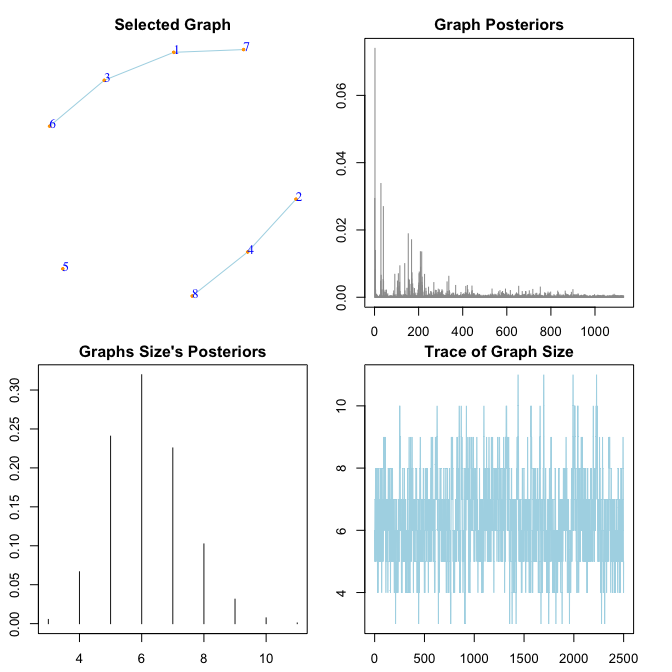$selected_g
[,1] [,2] [,3] [,4] [,5] [,6] [,7] [,8]
[1,]    0    0    1    0    0    0    1    0
[2,]    0    0    0    1    0    0    0    0
[3,]    0    0    0    0    0    1    0    0
[4,]    0    0    0    0    0    0    0    1
[5,]    0    0    0    0    0    0    0    0
[6,]    0    0    0    0    0    0    0    0
[7,]    0    0    0    0    0    0    0    0
[8,]    0    0    0    0    0    0    0    0

$p_links [,1] [,2] [,3] [,4] [,5] [,6] [,7] [,8] [1,] 0 0.24 1.00 0.03 0.06 0.18 0.69 0.03 [2,] 0 0.00 0.06 1.00 0.07 0.03 0.09 0.04 [3,] 0 0.00 0.00 0.05 0.09 0.56 0.28 0.06 [4,] 0 0.00 0.00 0.00 0.10 0.03 0.03 1.00 [5,] 0 0.00 0.00 0.00 0.00 0.08 0.13 0.03 [6,] 0 0.00 0.00 0.00 0.00 0.00 0.04 0.13 [7,] 0 0.00 0.00 0.00 0.00 0.00 0.00 0.03 [8,] 0 0.00 0.00 0.00 0.00 0.00 0.00 0.00$K_hat
[,1]  [,2]  [,3]  [,4]  [,5]  [,6]  [,7]  [,8]
[1,]  2.35  0.07  1.82  0.00  0.02  0.06 -0.31  0.00
[2,]  0.07  1.60 -0.01 -1.20 -0.01  0.00  0.02 -0.01
[3,]  1.82 -0.01  2.74 -0.01 -0.02 -0.26  0.11  0.01
[4,]  0.00 -1.20 -0.01  2.74 -0.03  0.00  0.00  1.02
[5,]  0.02 -0.01 -0.02 -0.03  0.98 -0.02  0.03 -0.01
[6,]  0.06  0.00 -0.26  0.00 -0.02  1.36  0.00 -0.03
[7,] -0.31  0.02  0.11  0.00  0.03  0.00  1.11  0.00
[8,]  0.00 -0.01  0.01  1.02 -0.01 -0.03  0.00  1.64

The summary results are the adjacency matrix of the selected graph (selected_g) based on BMA estimation, the estimated posterior probabilities of all possible links (p_links) and the estimated precision matrix (K_hat).

In addition, the function summary() reports a visualization summary of the results as we can see above. At the top-left is the graph with the highest posterior probability. The plot at the top-right gives the estimated posterior probabilities of all the graphs which are visited by the BDMCMC algorithm; it indicates that our algorithm visits more than $$2000$$ different graphs. The plot at the bottom-left gives the estimated posterior probabilities of the size of the graphs; it indicates that our algorithm visited mainly graphs with sizes between $$4$$ and $$18$$ links. At the bottom-right is the trace of our algorithm based on the size of the graphs.

The function compare() provides several measures to evaluate the performance of our algorithms and compare them with alternative approaches with respect to the true graph structure. To evaluate the performance of the BDMCMC algorithm and compare it with that of an alternative algorithm, we also run the RJMCMC algorithm under the same conditions as below.

sample.rjmcmc <- bdgraph( data = data.sim, method = "ggm", algorithm = "rjmcmc",
iter = 5000, save = TRUE, verbose = FALSE )

where the sampling algorithm from the joint posterior distribution is based on the RJMCMC algorithm.

Users can compare the performance of these two algorithms by using the code

plotroc( list( sample.bdmcmc, sample.rjmcmc ), data.sim, smooth = TRUE,
labels = c( "BDMCMC", "RJMCMC" ), color = c( "blue", "red" ) ) 

which visualizes an ROC plot for both algorithms, BDMCMC and RJMCMC.

We can also compare the performance of those algorithms by using the compare() function as follows:

compare( list( sample.bdmcmc, sample.rjmcmc ), data.sim,
main = c( "True graph", "BDMCMC", "RJMCMC" ), vis = TRUE )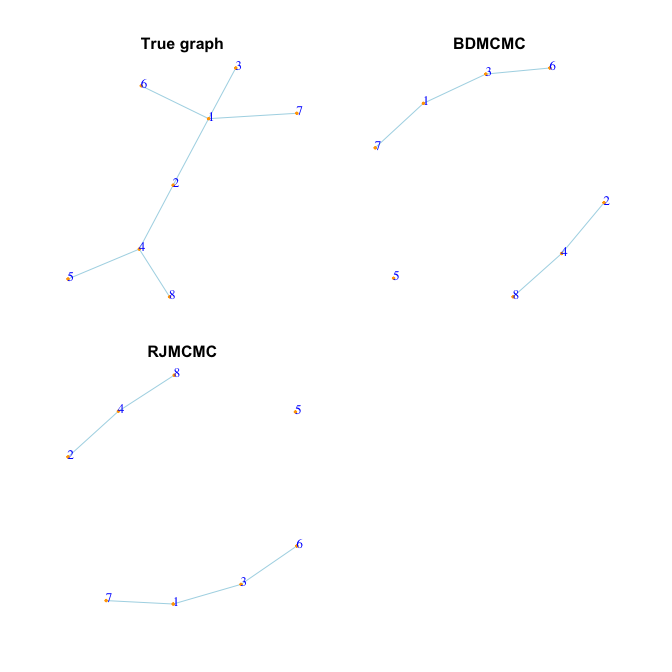True graph BDMCMC RJMCMC
True Positive           7  4.000  4.000
True Negative          21 20.000 20.000
False Positive          0  1.000  1.000
False Negative          0  3.000  3.000
F1-score                1  0.667  0.667
Specificity             1  0.952  0.952
Sensitivity             1  0.571  0.571
MCC                     1  0.592  0.592

The results show that for this specific simulated example both algorithms have more or less the same performance; See Mohammadi et al. (2021) for a comprehensive simulation study.

In this simulation example, we run both BDMCMC and RJMCMC algorithms for $$5,000$$ iterations, $$2,500$$ of them as burn-in. To check whether the number of iterations is enough and to monitoring the convergence of our both algorithm, we run

plotcoda( sample.bdmcmc, verbose = FALSE )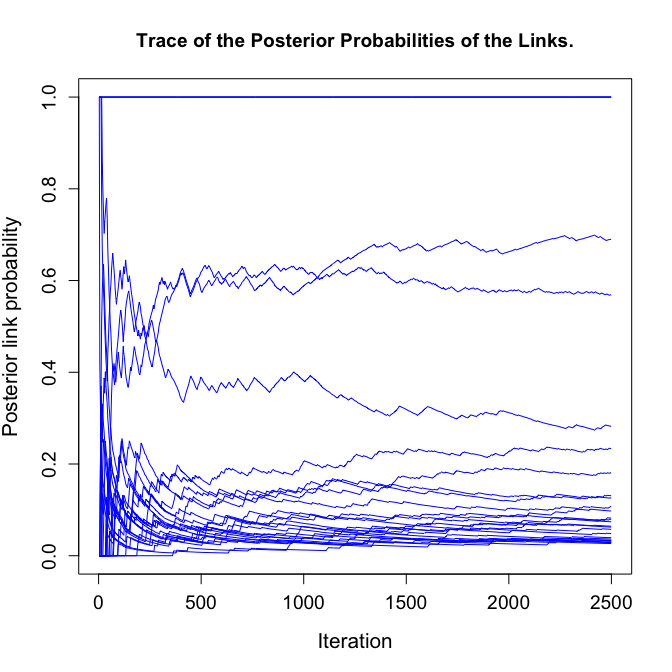plotcoda( sample.rjmcmc, verbose = FALSE )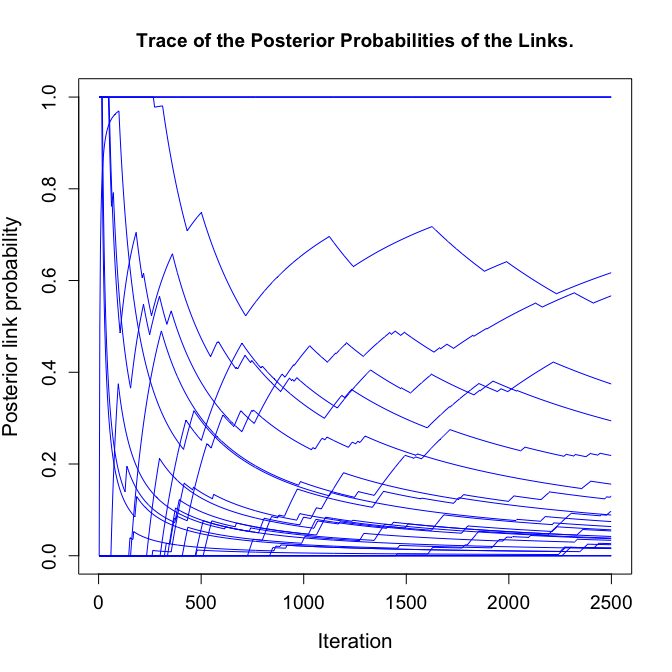The results indicate that the BDMCMC algorithm converges faster with compare with RJMCMC algorithm.